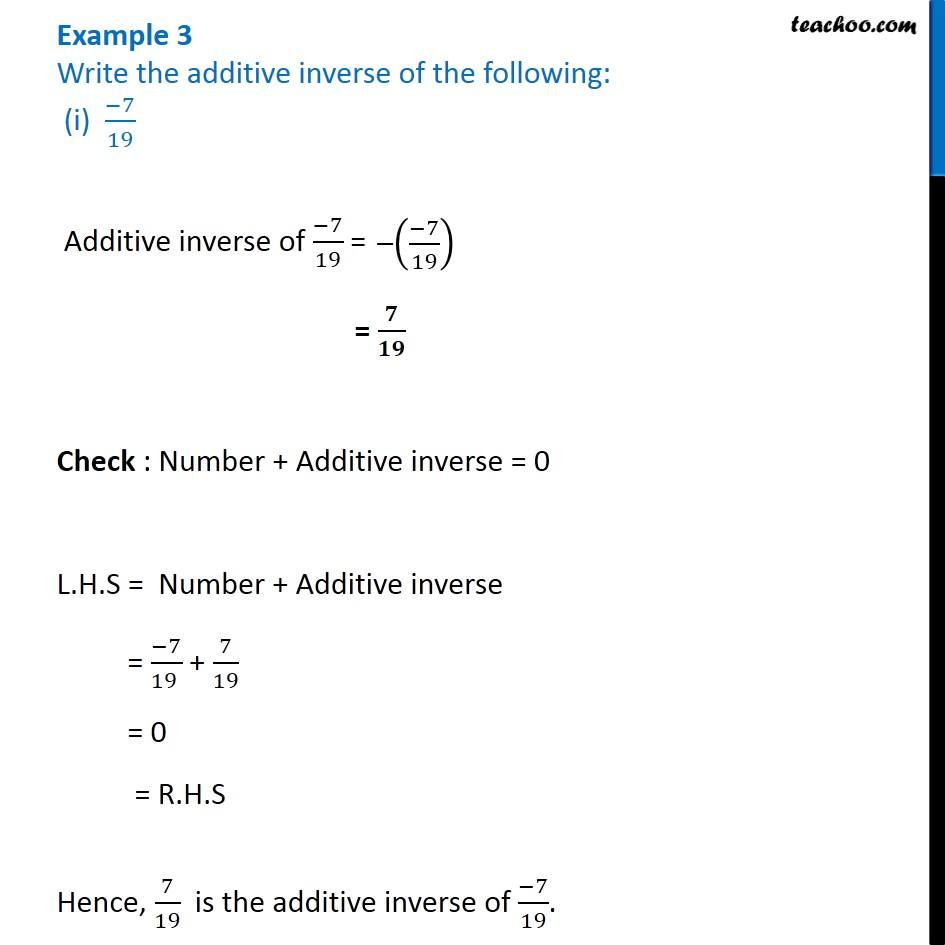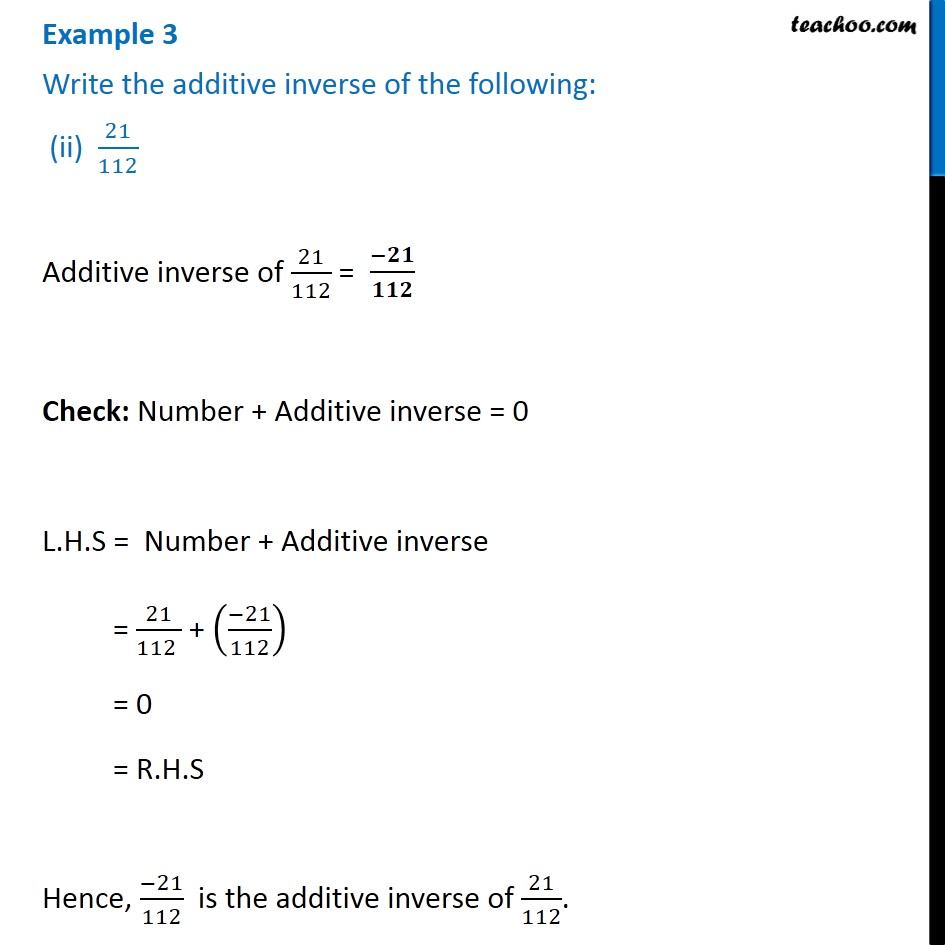Subscribe to our Youtube Channel - https://you.tube/teachoo

1. Chapter 1 Class 8 Rational Numbers
2. Serial order wise
3. Examples

Transcript

Example 3 Write the additive inverse of the following: (i) (−7)/19 Additive inverse of (−7)/19 = = 𝟕/𝟏𝟗 Check : Number + Additive inverse = 0 L.H.S = Number + Additive inverse = (−7)/(19 ) + 7/19 = 0 = R.H.S Hence, 7/19 is the additive inverse of (−7)/19. Example 3 Write the additive inverse of the following: (ii) 21/112 Additive inverse of 21/112 = Check: Number + Additive inverse = 0 L.H.S = Number + Additive inverse = 21/(112 ) + ((−21)/112) = 0 = R.H.S Hence, (−21)/112 is the additive inverse of 21/112.

Examples